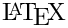Including formulas

Doxygen allows you to putformulas in the output (this works only for the HTML,and RTF output. To be able to include formulas (as images) in the HTML and RTF documentation, you will also need to have the following tools installed

• latex: thecompiler, needed to parse the formulas. To test I have used the teTeX 1.0 distribution.
• dvips: a tool to convert DVI files to PostScript files I have used version 5.92b from Radical Eye software for testing.
• gs: the GhostScript interpreter for converting PostScript files to bitmaps. I have used Aladdin GhostScript 8.0 for testing.

For the HTML output there is also an alternative solution using MathJax which does not require the above tools. If you enable USE_MATHJAX in the configuration then the latex formulas will be copied to the HTML "as is" and a client side javascript will parse them and turn them into (interactive) images.

There are four ways to include formulas in the documentation.

1. Using in-text formulas that appear in the running text. These formulas should be put between a pair of \f$commands, so  The distance between \f$(x_1,y_1)\f$and \f$(x_2,y_2)\f$is \f$\sqrt{(x_2-x_1)^2+(y_2-y_1)^2}\f$.  results in: The distance betweenandis. 2. Using in-text formulas that appear in the running text, contrary to \f$ it will not explicitly open the math mode in. These formulas should be put between \f( and \f) commands, so

  The LaTeX and Tex logos are: \f(\LaTeX \f) and \f(\TeX \f).


results in:

The LaTeX and Tex logos are:and.

3. Unnumbered displayed formulas that are centered on a separate line. These formulas should be put between \f[ and \f] commands. An example:
  \f[
|I_2|=\left| \int_{0}^T \psi(t)
\left\{
u(a,t)-
\int_{\gamma(t)}^a
\frac{d\theta}{k(\theta,t)}
\int_{a}^\theta c(\xi)u_t(\xi,t)\,d\xi
\right\} dt
\right|
\f]

results in:4. Formulas or other latex elements that are not in a math environment can be specified using \f{environment}, where environment is the name of theenvironment, the corresponding end command is \f}. Here is an example for an equation array
   \f{eqnarray*}{
g &=& \frac{Gm_2}{r^2} \\
&=& \frac{(6.673 \times 10^{-11}\,\mbox{m}^3\,\mbox{kg}^{-1}\,
\mbox{s}^{-2})(5.9736 \times 10^{24}\,\mbox{kg})}{(6371.01\,\mbox{km})^2} \\
&=& 9.82066032\,\mbox{m/s}^2
\f}

which results in:For the first and third commands one should make sure formulas contain valid commands in's math-mode. The second command should valid text-mode commands that also work in math-mode when using USE_MATHJAX. For the fourth command the section should contain valid command for the specific environment.

Warning
Currently, doxygen is not very fault tolerant in recovering from typos in formulas. It may be necessary to remove the files formula.repository that are written to the html, rtf etc. directories to get rid of an incorrect formula as well as the form_* files.

To have the possibility to define your owncommands, for e.g. formula building blocks or consistent writing of certain words, the configuration option FORMULA_MACROFILE can be used. to supply a file withcommands. This file can contain\newcommand and \renewcommand commands and they are included formulas (image version and MathJax version) as well as in the generatedoutput (for PDF generation).
The \newcommand (and \renewcommand) are restricted to a version without optional parameters so only the following types are supported:

\newcommand{\cmd}{replacement}
\newcommand{\cmd}[nr]{replacement}

e.g.

\newcommand{\E}{\mathrm{E}}
\newcommand{\ccSum}{\sum_{#1}^{#2}{#3}}